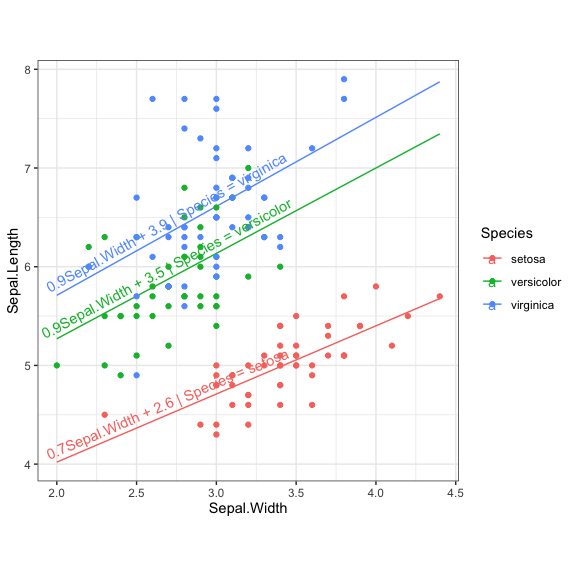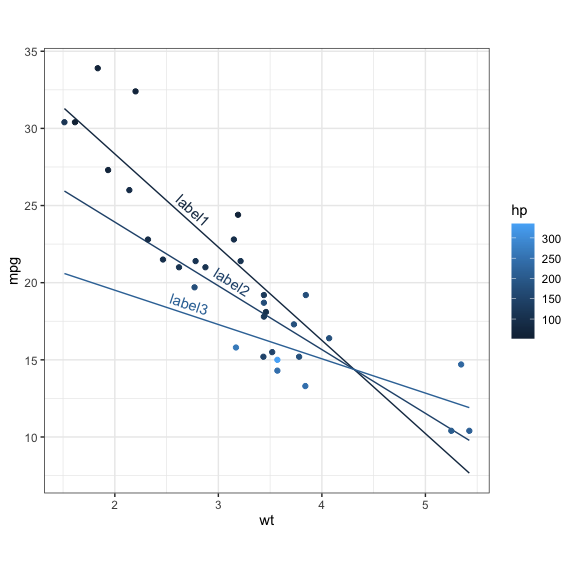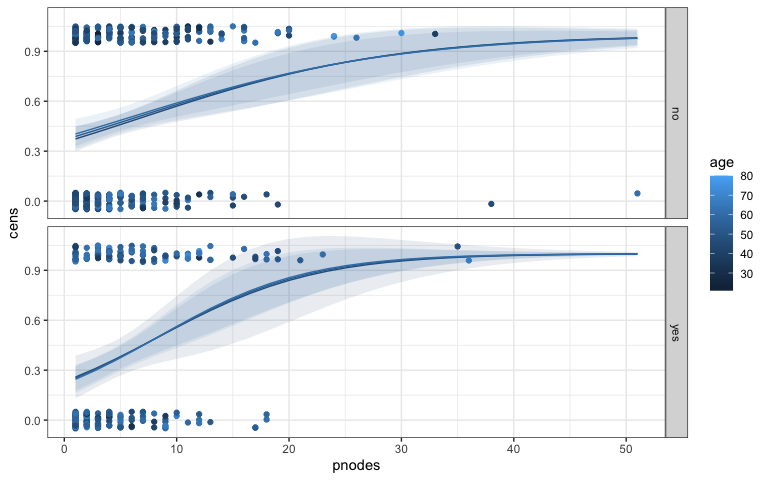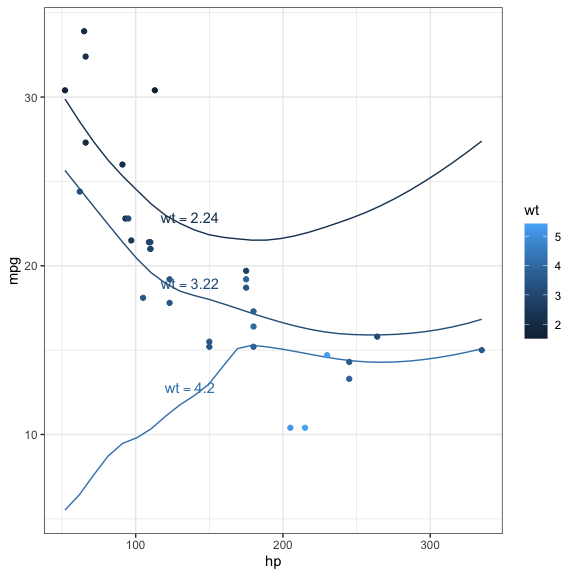# R package predict3d

#### 2023-04-11

R package predict3d aims to draw predicts plot for various regression models. The main two functions are ggPredict() for 2-dimensional plot and predict3d() for 3-dimensional plot.

## Package Installation

You can install the predict3d package from CRAN.

install.packages("predict3d")

You can install the developmental version of predict3d package from github.

if(!require(devtools)) install.packages("devtools")
devtools::install_github("cardiomoon/predict3d"")

## Linear Regression Models

You can draw linear regression models. First model has one categorical and one continuous explanatory variables.

require(predict3d)
require(rgl)
fit1=lm(Sepal.Length~Sepal.Width*Species,data=iris)
fit1

Call:
lm(formula = Sepal.Length ~ Sepal.Width * Species, data = iris)

Coefficients:
(Intercept)                    Sepal.Width
2.6390                         0.6905
Speciesversicolor               Speciesvirginica
0.9007                         1.2678
Sepal.Width:Speciesversicolor   Sepal.Width:Speciesvirginica
0.1746                         0.2110  

You can draw plot for this model. ggPredict() function draws a scatterplot with regression line and shows regression equations parallel to the regression lines.

ggPredict(fit1,digits=1)predict3d(fit1,radius=0.05)
Warning in Ops.factor(diff(y[!is.na(y)][1:2]), 0): '<' not meaningful for
factors
rglwidget(elementId = "1st")

Once you have create a model with predict3d(), you can rotate, zoom in and zoom out your object with your mouse.

The second model has two continuous variables as explanatory variables. You can change the labels and the relative x position and the y position.

fit2=lm(mpg~wt*hp,data=mtcars)
ggPredict(fit2,labels=paste0("label",1:3),xpos=c(0.3,0.4,0.3))predict3d(fit2,radius=3,palette=NULL,show.error=TRUE)
rglwidget(elementId = "2nd")

## Generalized Linear Models

You can draw generalized linear models.

require(TH.data)
fit3=glm(cens~pnodes*age*horTh,data=GBSG2,family=binomial)
ggPredict(fit3,se=TRUE,show.text = FALSE)predict3d(fit3,radius=0.5)
rglwidget(elementId = "3rd")

## Local Polynomial Regression Fitting

You can draw the loess model.

fit=loess(mpg~hp*wt,data=mtcars)
ggPredict(fit)predict3d(fit,radius=3)
rglwidget(elementId = "4th")

## Play with predict3d()

Once you have create a model with predict3d(), you can rotate your object with your mouse or R codes. For example, You can rotate you object with this R codes.

start <- proc.time()
while ((i <- 36*(proc.time() - start)) < 360) {
view3d(i, i/4);
}
play3d(spin3d(axis = c(1, 0, 0), rpm = 30), duration = 2)

You can save your 3d plot as a figure file or pdf file.

rgl.bringtotop()
rgl.snapshot("fig1.png")
rgl.postscript("fig2.pdf","pdf")

You can make movie file with the following R command. You can get movie.gif file.

movie3d(spin3d(axis = c(0, 0, 1)),dir=".", duration = 3,movie="movie")

You can export the plot into an interactive HTML file

writeWebGL(filename = "index.html")

For more information about package rgl, please read the package vignette at: https://CRAN.R-project.org/package=rgl/vignettes/rgl.html